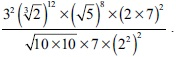# User ForumSubject :IMO    Class : Class 8

Find the number of prime factors ofA4
B7
C3
D5

the right answer for this should be 7, and not 3... could anyone explain why the answer for this is 3?

Class : Class 8

Class : Class 7

## Ans 3:

Class : Class 8
The answer is 3. When you solve the equation you get 3*3*1*1*2*14/1*1*1. Now we see that the prime numbers left are 3,3 and 2. Therefore there are 3 prime numbers.

Class : Class 9
4

Class : Class 4

## Ans 6:

Class : Class 6
the solution of the equation is 3*3*5*5*5*2*7 which has seven prime factors

## Ans 7:

Class : Class 8
The answer should be option (A) = 4. There are 4 prime factors, i.e. 2, 3, 5 and 7. The solution contradicts the question's answer.

Class : Class 9

## Ans 9:

Class : Class 8
Yes .... The answer is 4. ( 2, 3 ,5 and 7)

Class : Class 8
ans is 7

## Ans 11:

Class : Class 8
The answer is 4. There are 4 prime factors: 2,3,5 and 7

## Ans 12:

Class : Class 8
The answer is 3. When you solve the equation you get 3*3*1*1*2*14/1*1*1. Now we see that the prime numbers left are 3,3 and 2. Therefore there are 3 prime numbers.